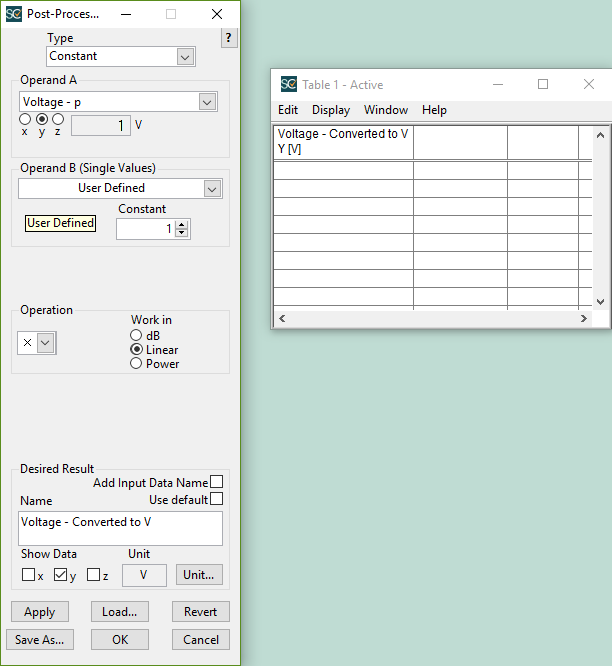# How Do I Change Units In SoundCheck?

Units can be changed in two different ways in SoundCheck: Directly from the memory list or as a post processing step in a sequence.

To change units directly from the memory list, right click on the item and select “Units”.

A value “Voltage” in the memory list of 0dBV: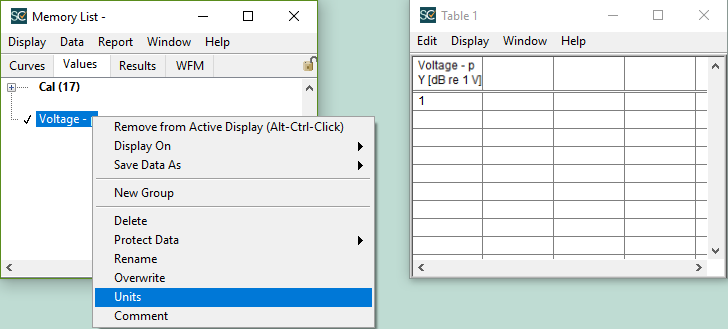There will be an option to select log or linear.

If you select log there are some common logarithmic units including dBSPL, dBm and dBPA as well as the option to set a custom dB Reference value and unit.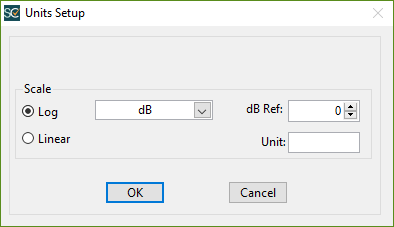Linear gives the option to change the unit.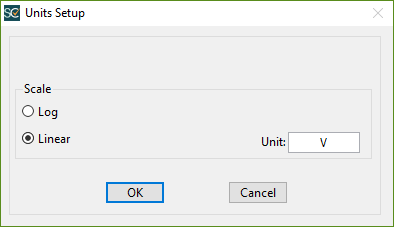The unit of “Voltage” has been changed to linear volts, giving a value of 1V from 0dBV.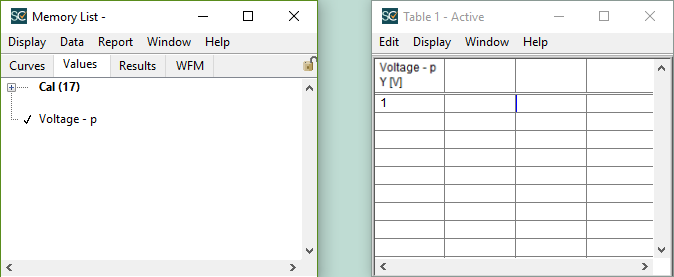If you want the values to be changed directly in a sequence, you can set up a post processing sequence to multiply by a constant of one. There is an option to change units in the post processing step that has the same interface as the option in the memory list.

This is the previous “Voltage” value of 0dBV multiplied by 1 with the result units changed to linear volts: